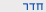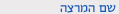שנה"ל תשע"האותות אקראיים ורעש
Random Signals and Noise
0512-3632-02
הנדסה | תואר ראשון - חשמל ואלקטרוניקהסמ'  א' 1200-1400 'ה 406 ולפסון הנדסה תרגיל מר אורדנטליך אור סמ'  א' תרגיל גב' אלאור הדר מר טרידנסקי סרגיי
ש"ס:  2.0

סילבוס מקוצר
שעות:                5 ש"ס
משקל:                4
דרישות קדם: מבוא להסתברות וסטטיסטיקה, מבוא לניתוח אותות
חלק א': משתנים אקראיים ופעולות עליהם
1. הסתברות ומשתנים אקראיים (כשבועיים)
* חזרה על מושגי יסוד: מוטיבציה הנדסית, מרחב הסתברות, אקסיומות
* חזרה על מושגי יסוד עבור משתנה אקראי יחיד
* פונקציה אופיינית, מומנטים
* פונקציות של משתנים אקראיים

2. ווקטורים אקראיים (כשבועיים)
* שני משתנים אקראיים: פילוג משותף, מותנה ושולי
* וקטור של משתנים אקראיים
* וקטור גאוסי

3. שערוך (כשבועיים)
* שיערוך אופטימלי, קריטריוני שגיאה
* שערוך במובן של שגיאה ריבועית ממוצעת מינימלית (MMSE)
* שיערוך לינארי אופטימלי (LMMSE)

חלק ב': תהליכים אקראיים ופעולות עליהם
4. מבוא, הגדרות ותכונות (כשבוע)
* איך נוצר תהליך אקראי? (פרמטר אקראי, תהליך i.i.d., הגדרה רקורסיבית)
* פילוג משותף, פונקציית האוטוקורלציה
* סטאציונריות במובן הצר ( SSS) והרחב (WSS)

5. תהליכים בסיסיים בזמן בדיד ובזמן רציף (כשבוע)
* תהליך אוטו-רגרסיבי (תנאים לסטאציונריות, מרקוביות)
* צעד אקראי, רעש לבן בזמן בדיד
* תהליך אקראי גאוסי
* תהליך Wiener / תנועת Brown

6. שרשראות מרקוב (כשבוע)
* מטריצת מעבר, פילוג סטציונארי
* אפיון שרשראות לפי גרף המצבים

7. ארגודיות (כשבוע)
* חוק המספרים הגדולים
* ארגודיות בתוחלת ובקורלציה

8. ספקטרום הספק (כשבוע)
* הגדרות, פריודוגראמה, רעש לבן בזמן רציף

9. מעבר תהליכים WSS במערכות לינאריות (כשבועיים)
* סטאציונאריות משותפת של תהליכים אקראיים
* מעבר תהליך אקראי דרך מערכת לינארית
* שיערוך לינארי אופטימלי במובן MSE (מסנן וינר)
* מערכות מרובות כניסות ויציאות
* עיבוד מקבילי של פסי תדר.

10. תהליכים אקראיים מתקדמים (כשבוע)
* תהליך אקראי Poisson

Course description
Credit points: 4
Prerequisites; Introductiot to Probability and Statistics;Introduction to Signal Analysis.

1. Problem sets will be posted once a week. The solution will be posted a week after.

2. Two midterms will be given during the semester. The problems will be based on ones that appeared in the lectures, recitations and HW assignments.
3. During the course we will publish up to four Matlab assignments which are related to the topics covered in class. The submission of these exercises will be done in pairs, and is accompanied by an oral exam on the exercises.
4. A Final exam which covers all the course's material.

Part A: Random Variables and Operations
1. Probability and Random Variables (about two weeks)
• Engineering motivation, probability space, axioms.
• Revisiting basic concepts for a single random variable.
• The characteristic function, moments.
• Functions of random variables.

2. Random vectors (about two weeks)
• Two random variables: joint, conditional and marginal distributions.
• Vectors of random variables.
• Gaussian vectors.

• Optimal estimation, error criteria.
• Minimum mean squared-error (MMSE) estimation.
• Linear minimum mean squared-error (LMMSE) estimation.

Part B: Random Processes
4. Introduction, definitions and properties. (about one week)
• The formation of random processes.
• Joint distribution, autocorrelation function.
• Strict-sense and wide-sense stationarity (SSS and WSS).

5. Basic discrete –time and continuous-time processes (about one week)
• Autoregressive process (stationarity conditions, Markovity).
• Random walk, discrete-time white-noise.
• Gaussian random processes.
• Wiener process \ Brownian motion.

6. Markov chains (about one week)
• Transition matrix, stationary distribution.
• Characterizing a Markov chains its state diagram.

• The law of large numbers.
• Mean-ergodicity, correlation-ergodicity.

8. Power spectrum density (about one week)
• Definitions, periodogram, continuous-time white-noise.

9. Linear time-invariant (LTI) processing of WSS processes (about two weeks)
• Joint stationarity of random processes.
• Random processes passing LTI systems.
• Optimal linear MMSE estimation (Wiener filtering).
• Multiple-input multiple-output (MIMO) systems.
• Parallel processing of frequency-bands.